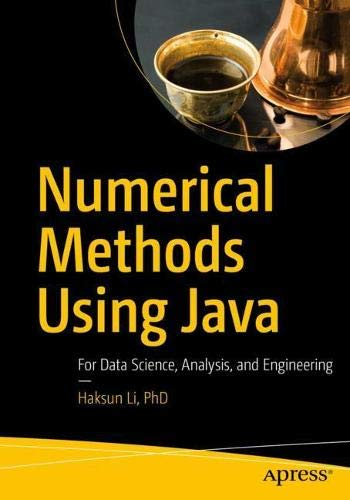# Numerical Methods Using Java: For Data Science, Analysis, and Engineering

• Length: 1200 pages
• Edition: 1
• Publisher:
• Publication Date: 2022-01-30
• ISBN-10: 1484267966
• ISBN-13: 9781484267967
Description

Implement numerical algorithms in Java using NM Dev, an object-oriented and high-performance programming library for mathematics.You’ll see how it can help you easily create a solution for your complex engineering problem by quickly putting together classes.

Numerical Methods Using Java covers a wide range of topics, including chapters on linear algebra, root finding, curve fitting, differentiation and integration, solving differential equations, random numbers and simulation, a whole suite of unconstrained and constrained optimization algorithms, statistics, regression and time series analysis. The mathematical concepts behind the algorithms are clearly explained, with plenty of code examples and illustrations to help even beginners get started.

What You Will Learn

• Program in Java using a high-performance numerical library
• Learn the mathematics for a wide range of numerical computing algorithms
• Convert ideas and equations into code
• Put together algorithms and classes to build your own engineering solution
• Build solvers for industrial optimization problems
• Do data analysis using basic and advanced statistics

Who This Book Is For

Programmers, data scientists, and analysts with prior experience with programming in any language, especially Java.